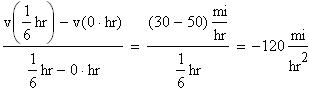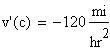Problem
At 7 p.m., a car is traveling at 50 miles per hour. Ten minutes later, the car has slowed to 30 miles per hour. Show that at some time between 7 and 7:10 the car's acceleration is exactly 120, in units of miles per hours squared.
Solution
Let v(t) be the car's velocity, where t is the elapsed hours since 7 p.m. Then v'(t) is a function describing the car's acceleration at time t. Remember that 10 minutes equals one sixth of an hour. Then the average acceleration of the car between 7 and 7:10 can be writtenBy the Mean Value Theorem, there is some time c in the interval [0, 1/6] such that